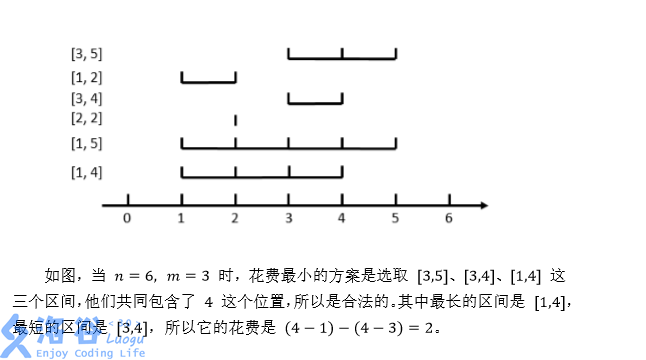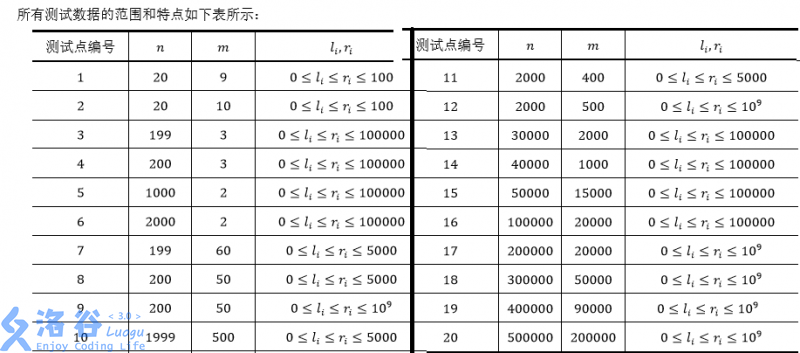# P1712 [NOI2016]区间

• 1.2K通过
• 3.6K提交
• 题目提供者 kkksc03 吉祥物
• 评测方式 云端评测
• 标签 排序 离散化 线段树 NOI系列 2016 O2优化 高性能
• 难度 省选/NOI-
• 时空限制 1000ms-3000ms / 256MB
• 提示：收藏到任务计划后，可在首页查看。

## 题目描述

在数轴上有$N$ 个闭区间 $[l_1,r_1],[l_2,r_2],...,[l_n,r_n]$ 。现在要从中选出$M$ 个区间，使得这$M$ 个区间共同包含至少一个位置。换句话说，就是使得存在一个 $x$ ，使得对于每一个被选中的区间$[l_i,r_i]$ ，都有 $l_i≤x≤r_i$ 。

对于一个合法的选取方案，它的花费为被选中的最长区间长度减去被选中的最短区间长度。区间$[l_i,r_i]$ 的长度定义为$r_i-l_i$ ，即等于它的右端点的值减去左端点的值。

求所有合法方案中最小的花费。如果不存在合法的方案，输出$-1$ 。

## 输入输出格式

输入格式：

第一行包含两个正整数$N,M$ 用空格隔开，意义如上文所述。保证$1≤M≤N$

接下来$N$ 行，每行表示一个区间，包含用空格隔开的两个整数$l_i$ 和$r_i$ 为该区间的左右端点。

$N<=500000,M<=200000,0≤li≤ri≤10^9$

输出格式：

只有一行，包含一个正整数，即最小花费。

## 输入输出样例

输入样例#1： 复制
6 3
3 5
1 2
3 4
2 2
1 5
1 4
输出样例#1： 复制
2

## 说明提示
标程仅供做题后或实在无思路时参考。
请自觉、自律地使用该功能并请对自己的学习负责。
如果发现恶意抄袭标程，将按照I类违反进行处理。